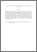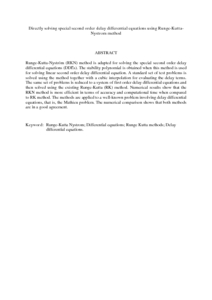# Directly solving special second order delay differential equations using Runge-Kutta-Nystrom method

## Citation

Mechee, Mohammed and Ismail, Fudziah and Senu, Norazak and Siri, Zailan (2013) Directly solving special second order delay differential equations using Runge-Kutta-Nystrom method. Mathematical Problems in Engineering, 2013 (830317). pp. 1-7. ISSN 1024-123X

## Abstract

Runge-Kutta-Nyström (RKN) method is adapted for solving the special second order delay differential equations (DDEs). The stability polynomial is obtained when this method is used for solving linear second order delay differential equation. A standard set of test problems is solved using the method together with a cubic interpolation for evaluating the delay terms. The same set of problems is reduced to a system of first order delay differential equations and then solved using the existing Runge-Kutta (RK) method. Numerical results show that the RKN method is more efficient in terms of accuracy and computational time when compared to RK method. The methods are applied to a well-known problem involving delay differential equations, that is, the Mathieu problem. The numerical comparison shows that both methods are in a good agreement.Preview
PDF (Abstract)
Directly solving special second order delay differential equations using Runge.pdfView Item